Courses

# Test: Time & Work (Hard)

## 30 Questions MCQ Test Quantitative Aptitude for Banking Preparation | Test: Time & Work (Hard)

Description
This mock test of Test: Time & Work (Hard) for Banking Exams helps you for every Banking Exams entrance exam. This contains 30 Multiple Choice Questions for Banking Exams Test: Time & Work (Hard) (mcq) to study with solutions a complete question bank. The solved questions answers in this Test: Time & Work (Hard) quiz give you a good mix of easy questions and tough questions. Banking Exams students definitely take this Test: Time & Work (Hard) exercise for a better result in the exam. You can find other Test: Time & Work (Hard) extra questions, long questions & short questions for Banking Exams on EduRev as well by searching above.
QUESTION: 1

### A and B can do a piece of work in 30 days, while B and C can do the same work in 24 days and C and A in 20 days. They all work together for 10 days when B and C leave. How many days more will A take to finish the work?

Solution:

2(A + B + C)'s 1 clay's work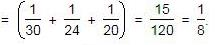Therefore. (A + B + C)'s 1 clay's work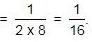Work done by A, B. C in 10 days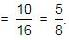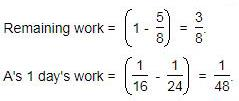Now, 1/48 work is done by A in 1 day.
So. 3/8 work will be done by A in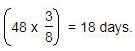QUESTION: 2

### Ravi and Kumar are working on an assignment. Ravi takes 6 hours to type 32 pages on a computer, while Kumar takes 5 hours to type 40 pages. How much time will they take, working together on two different computers to type an assignment of 110 pages?

Solution: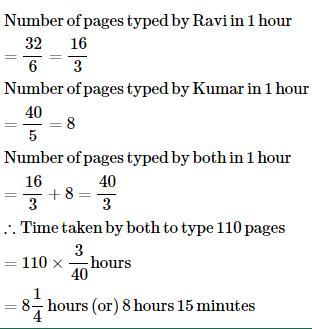QUESTION: 3

### A alone can do a piece of work in 6 days and B alone in 8 days. A and B undertook to do it for Rs. 3200. With the help of C, they completed the work in 3 days. How much is to be paid to C?

Solution:

C's 1 day's work = 1/3 - (1/6 + 1/8)
= 1/3 - 7/24
= 1/24
A's wages : B's wages : C's wages = 1/6 : 1/8 : 1/24
=> 4 : 3 : 1
C's share (for 3 days) = Rs.(3 * 1/24 * 3200)
= Rs. 400

QUESTION: 4

A can lay railway track between two given stations in 16 days and B can do the same job in 12 days. With help of C, they did the job in 4 days only. Then, C alone can do the job in:

Solution:
QUESTION: 5

A can do a work in 15 days and B in 20 days. If they work on it together for 4 days, then the fraction of the work that is left is :

Solution:

A can do work in 15 days therefore amount of work done by A in 1 day is 60/15 = 4 units
B can do work in 15 days therefore amount of work done by A in 1 day is 60/20 = 3 units
Total amount of work done in 1 day =A work of 1 day+B's work of 1 day=4 units +3 units=7 units
Amount of work done in 4 days =4*7=28 units
Amount of Work Left = 60–28=32 units
Fraction of work left = Amount of work left/ Total Work = 32/60
= 8/15.

QUESTION: 6

A and B can complete a work in 15 days and 10 days respectively. They started doing the work together but after 2 days B had to leave and A alone completed the remaining work. The whole work was completed in :

Solution:

Let total work be 30 units (LCM of 10 and 15).
In one day, A can do 2 units of work and B can do 3 units of work.
In one day, both A and B can do 5 units of work.
In two days, A and B will complete 10 units of work. Remaining 20 units can be completed by A in 10 days (at rate of 2 units per day).
Hence, whole work will be completed in 12 days.

QUESTION: 7

4 men and 6 women can complete a work in 8 days, while 3 men and 7 women can complete it in 10 days. In how many days will 10 women complete it?

Solution:

Let 1 man's 1 day's work = x and 1 woman's 1 day's work = y.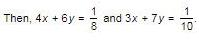Solving the two equations, we get.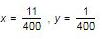∴ 1 woman's 1 day's work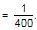⇒ 10 women's 1 day's work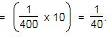Hence. 10 women will complete the work in 40 days

QUESTION: 8

A, B and C can complete a piece of work in 24, 6 and 12 days respectively. Working together, they will complete the same work in:

Solution:

A’s 1 day work = 1/24
B’s 1 day work = 1/6
C’s 1 day work = 1/12
(A+B+C)’s 1 day work = 1/24 + 1/6 + 1/12 = 7/24
The work will be completed by them is 24/7 days.

QUESTION: 9

X and Y can do a piece of work in 20 days and 12 days respectively. X started the work alone and then after 4 days Y joined him till the completion of the work. How long did the work last?

Solution:
QUESTION: 10

If A and B together can complete a piece of work in 15 days and B alone in 20 days, in how many days can A alone complete the work?

Solution:

(A + B)’s 1 day’s work = 1/15
B’s 1 day’s work  = 1/20

∴ A’s 1 day’s work
= 1/15 - 1/20
= (4-3)/60
= 1/60

∴ A alone will do the work in 60 days.

QUESTION: 11

A can do a piece of work in 4 hours; B and C together can do it in 3 hours, while A and C together can do it in 2 hours. How long will B alone take to do it?

Solution:

A’s 1 hour work = 1/4
(B + C)’s 1 hour work = 1/3
(C + A)’s 1 hour work = 1/2
(A + B + C)’s 1 hour work = 1/4 + 1/3 =7/12
B’s 1 hour work = 7/12 - 1/2 = 1/12
B alone can do a piece of work in 12 hours.

QUESTION: 12

A can finish a work in 18 days and B can do the same work in 15 days. B worked for 10 days and left the job. In how many days, A alone can finish the remaining work?

Solution:

{ A can finish work in 18 days.
It means A can do the work in one day=  1 / 18 part
B can finish work in 15 days.
It means B can do the work in one day =  1 / 15 part
B worked alone for 10 days and then left the job
Thus in 10 days B has completed  part of work:-
1 / 15* 10=  10 / 15 part  or  2 / 3 part of work.
Remaining work left = ( 1- 2 / 3 )
=  1 / 3 part of work remaining
A can do 1 / 18 part of work in one day
Therefore, 1 / 3 part will be completed by him
=  1 / 1/18 * ⅓
= 1 /3 * 18 / 1
= 18 / 3 days
= 6 days
( A alone will complete the work in 6 days)

QUESTION: 13

A works twice as fast as B. If B can complete a work in 12 days independently, the number of days in which A and B can together finish the work in

Solution: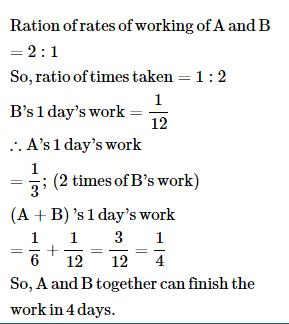QUESTION: 14

A and B together can do a piece of work in 30 days. A having worked for 16 days, B finishes the remaining work alone in 44 days. In how many days shall B finish the whole work alone?

Solution:
QUESTION: 15

A can do a certain work in the same time in which B and C together can do it. If A and B together could do it in 10 days and C alone in 50 days, then B alone could do it in:

Solution:

Let a, b and c be the fraction of work per day by A,B & C respectively.
c = 1/50
a + b = 1/10 = 5/50 .
a = b+ c.
Therefore,
2b + c = 5/50
2b = 5/50 - 1/50 = 2/25
b = 1/25
B will do it in 25 days.

QUESTION: 16

A machine P can print one lakh books in 8 hours, machine Q can print the same number of books in 10 hours while machine R can print them in 12 hours. All the machines are started at 9 A.M. while machine P is closed at 11 A.M. and the remaining two machines complete work. Approximately at what time will the work (to print one lakh books) be finished ?

Solution:
QUESTION: 17

P can complete a work in 12 days working 8 hours a day. Q can complete the same work in 8 days working 10 hours a day. If both P and Q work together, working 8 hours a day, in how many days can they complete the work?

Solution:
QUESTION: 18

A and B can do a work in 8 days, B and C can do the same work in 12 days. A, B and C together can finish it in 6 days. A and C together will do it in :

Solution:

(A + B + C)'s 1 day's work = 1/6
(A + B)'s 1 day's work = 1/8
(B + C)'s 1 day's work = 1/12
Therefore (A + C)'s 1 day's work
= (2 x 1/6) - (1/8 + 1/12)
= (1/3 - 5/24)
= 3/24
= 1/8
So, A and C together will do the work in 8 days.

QUESTION: 19

A can finish a work in 24 days, B in 9 days and C in 12 days. B and C start the work but are forced to leave after 3 days. The remaining work was done by A in:

Solution:

(B + C)'s 1 day's work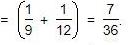Work done by B and C in 3 days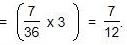Remaining work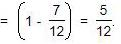Now, 1/24 work is done by A in 1 day.
So, 5/12 work is done by A in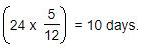QUESTION: 20

If 6 men and 8 boys can do a piece of work in 10 days while 26 men and 48 boys can do the same in 2 days, the time taken by 15 men and 20 boys in doing the same type of work will be:

Solution:
QUESTION: 21

A is thrice as good as workman as B and therefore is able to finish a job in 60 days less than B. Working together, they can do it in:

Solution:
QUESTION: 22

A does 80% of a work in 20 days. He then calls in B and they together finish the remaining work in 3 days. How long B alone would take to do the whole work?

Solution:
QUESTION: 23

A takes twice as much time as B or thrice as much time as C to finish a piece of work. Working together, they can finish the work in 2 days. B can do the work alone in:

Solution:
QUESTION: 24

Sakshi can do a piece of work in 20 days. Tanya is 25% more efficient than Sakshi. The number of days taken by Tanya to do the same piece of work is:

Solution:
QUESTION: 25

A is 30% more efficient than B. How much time will they, working together, take to complete a job which A alone could have done in 23 days?

Solution:

Ratio of times taken by A and B
= 100 :130
= 10:13
Suppose B takes x days to do the work
Then,10:13::23:x
⇒x = (23×13)/10
⇒x = 299/10
A's 1day's work = 1/23
B's 1day's work = 10/299
(A + B)'s 1 day's work = 1/23 + 10/299 =23/299 = 1/13
∴ A and B together can complete the work in 13 days.

QUESTION: 26

X can do a piece of work in 40 days. He works at it for 8 days and then Y finished it in 16 days. How long will they together take to complete the work?

Solution:

No of days taken by X to finish the work= 40
So part of work done by X in 1 day= 1/40
Part of work done by X in 8 days= 8/40 = 1/5
The remaining part = 1 - 1/5 = 4/5
No of days taken by Y to finish 4/5 part = 16
Part of work done by Y in 1 day = (4/5)/16
= (4/5)(1/16)
= 1/20
Part of work done by both together in 1 day = (1/40)+(1/20)= 3/40
Hence, X and Y will together complete the work in = 40/3

QUESTION: 27

A, B and C can do a piece of work in 20, 30 and 60 days respectively. In how many days can A do the work if he is assisted by B and C on every third day?

Solution:
QUESTION: 28

10 women can complete a work in 7 days and 10 children take 14 days to complete the work. How many days will 5 women and 10 children take to complete the work?

Solution:
QUESTION: 29

A and B can together finish a work 30 days. They worked together for 20 days and then B left. After another 20 days, A finished the remaining work. In how many days A alone can finish the work?

Solution:
QUESTION: 30

Twenty women can do a work in sixteen days. Sixteen men can complete the same work in fifteen days. What is the ratio between the capacity of a man and a woman?

Solution: